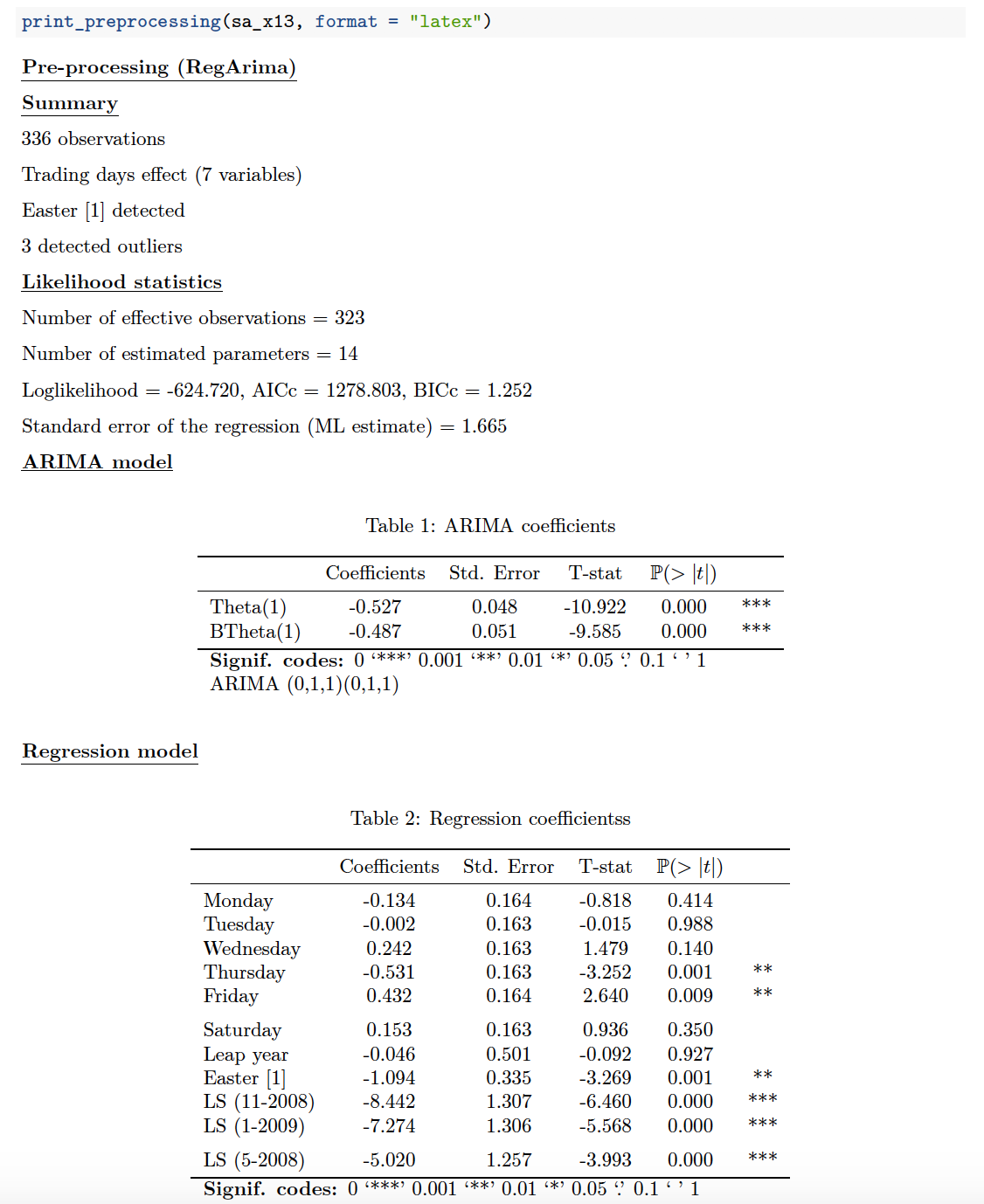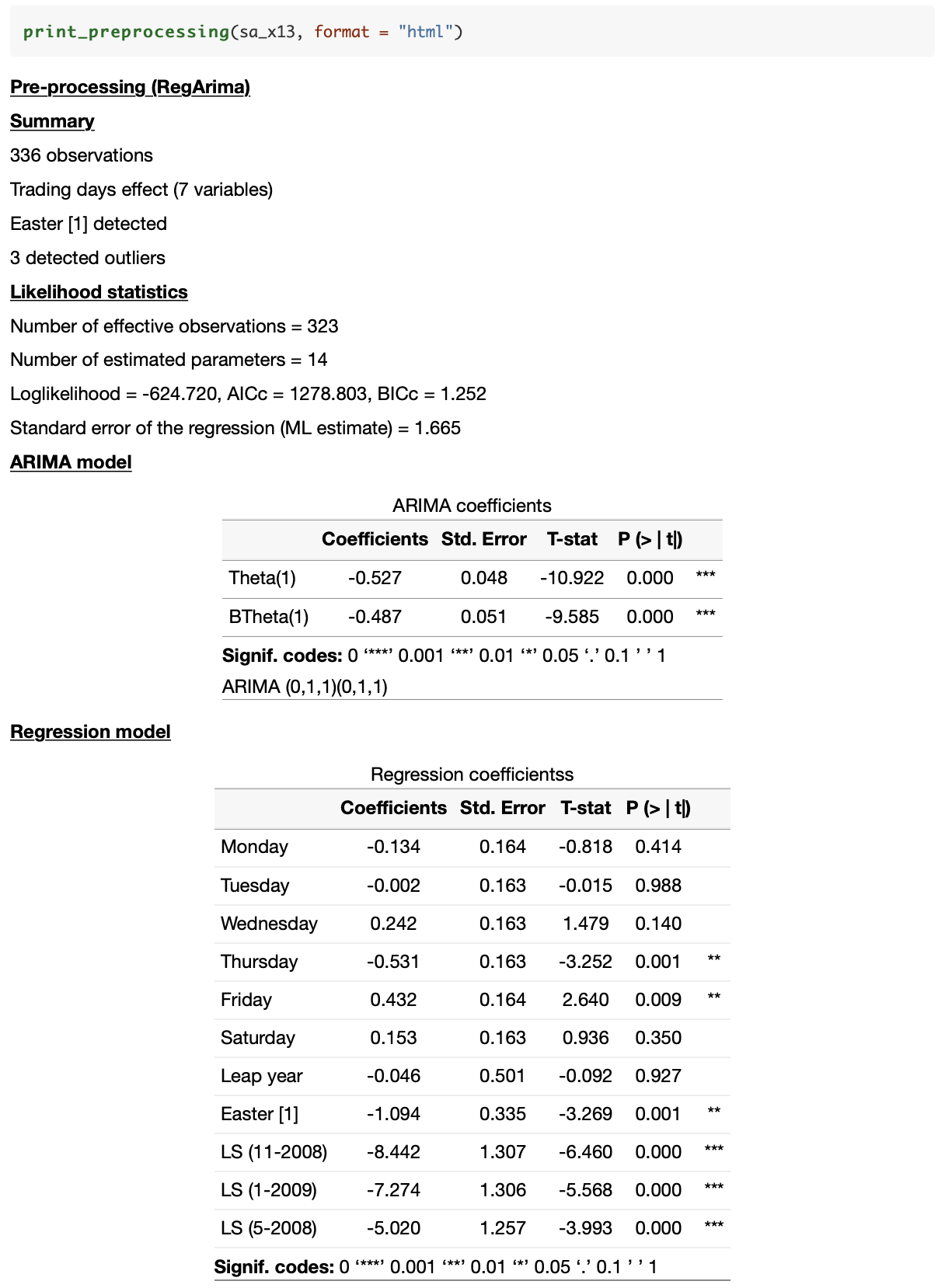# rjdmarkdown

## Overview

rjdmarkdown gives a set of functions to print nicely your models created by RJDemetra.

## Installation

rjdmarkdown relies on RJDemetra that requires Java SE 8 or later version.

``````# Install release version from CRAN
install.packages("rjdmarkdown")

# Install development version from GitHub
# install.packages("devtools")
devtools::install_github("AQLT/rjdmarkdown")``````

## Usage

The functions developped are:

• `print_preprocessing()` for the pre-processing model;
• `print_decomposition()` for the decomposition;
• `print_diagnostics()` to print diagnostics tests on the quality of the seasonal adjustment.

The available outputs are LaTeX (`format = "latex"`, the package booktabs and float must be used) and HTML outputs (`format = "html"`).

The option `results='asis'` must be used in the chunk:

``````library(RJDemetra)
library(rjdmarkdown)
mysa <- x13(ipi_c_eu[, "FR"])
print_preprocessing(mysa, format = "latex")
print_decomposition(mysa, format = "latex")
print_diagnostics(mysa, format = "latex")``````

A R Markdown can also directly be created and render with the `create_rmd` function. It can take as argument a `SA`, `jSA`, `sa_item`, `multiprocessing` (all the models of the `multiprocessing` are printed) or workspace object (all the models of all the `multiprocessing` of the `workspace` are printed). For example:

``````sa_ts <- tramoseats(ipi_c_eu[, "FR"])
wk <- new_workspace()
new_multiprocessing(wk, "sa1")
# It's important to compute the workspace to be able to import the models
compute(wk)

output_file <- tempfile(fileext = ".Rmd")
create_rmd(wk, output_file,
output_format = c("pdf_document", "html_document"),
output_options = list(toc = TRUE,
number_sections = TRUE))
# To open the file:
browseURL(sub(".Rmd",".pdf", output_file, fixed = TRUE))
browseURL(sub(".Rmd",".html", output_file, fixed = TRUE))``````

### LaTeX output### HTML output